20-2 嚙踝蕭嚙踝蕭嚙踝蕭嚙踝蕭嚙踝蕭嚙踝蕭嚙踝蕭嚙?

Example 1: 20-音訊讀寫、錄製與播放/audioRead01.m[y, fs]=audioread('welcome.wav'); sound(y, fs); % 播放此音訊 time=(1:length(y))/fs; % 時間軸的向量 plot(time, y); % 畫出時間軸上的波形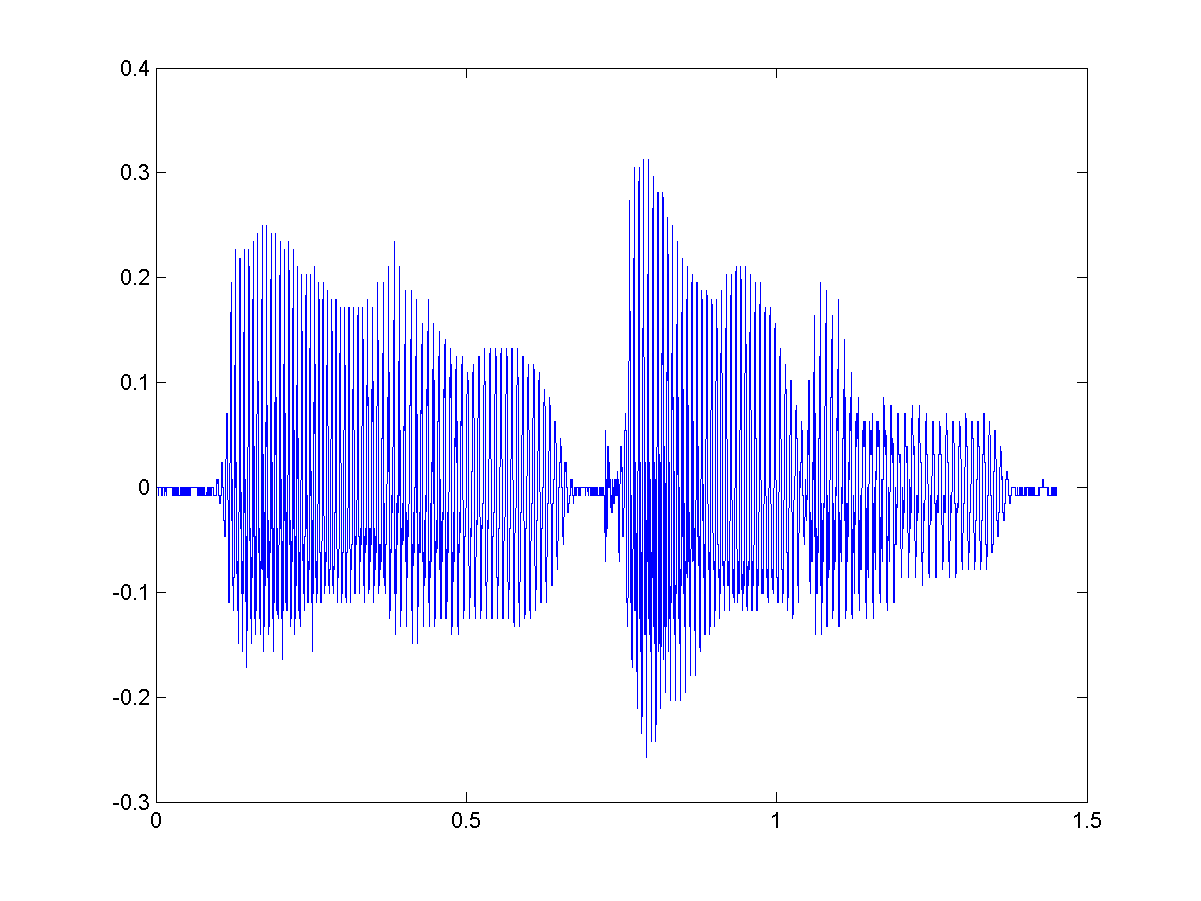Hint

Example 2: 20-音訊讀寫、錄製與播放/audioInfo01.mfileName='welcome.wav'; info=audioinfo(fileName); fprintf('檔案名稱 = %s\n', info.Filename); fprintf('壓縮方式 = %s\n', info.CompressionMethod); fprintf('通道個數 = %g 個\n', info.NumChannels); fprintf('取樣頻率 = %g Hz\n', info.SampleRate); fprintf('取樣點總個數 = %g 個\n', info.TotalSamples); fprintf('音訊長度 = %g 秒\n', info.Duration); fprintf('取樣點解析度 = %g 位元/取樣點\n', info.BitsPerSample); 檔案名稱 = D:\users\jang\books\matlabProgramming4beginner\example\20-音訊讀寫、錄製與播放\welcome.wav 壓縮方式 = Uncompressed 通道個數 = 1 個 取樣頻率 = 11025 Hz 取樣點總個數 = 16001 個 音訊長度 = 1.45134 秒 取樣點解析度 = 8 位元/取樣點

Hint

Example 3: 20-音訊讀寫、錄製與播放/audioRead03.mfileName='welcome.wav'; [y, fs]=audioread(fileName); info=audioinfo(fileName); nbits=info.BitsPerSample; y0=y*(2^nbits/2)+(2^nbits/2); % y0 是原先儲存在音訊檔案中的值 difference=sum(abs(y0-round(y0))) difference = 0

MATLAB 對於音檔資料的正規化方式，可以列表如下：

Unsigned 8-bit integer (uint8) y $\in$ [0, $2^8-1$] (y-$2^7$)/$2^7$
Signed 16-bit integer (int16) y $\in$ [$-2^{15}$, $2^{15}-1$] y/$2^{15}$
Signed 32-bit integer (int32) y $\in$ [$-2^{31}$, $2^{31}-1$] y/$2^{31}$

Example 4: 20-音訊讀寫、錄製與播放/audioRead04.mfileName='flanger.wav'; [y, fs]=audioread(fileName); % 讀取音訊檔 sound(y, fs); % 播放音訊 left=y(:,1); % 左聲道音訊 right=y(:,2); % 右聲道音訊 subplot(2,1,1), plot((1:length(left))/fs, left); subplot(2,1,2), plot((1:length(right))/fs, right);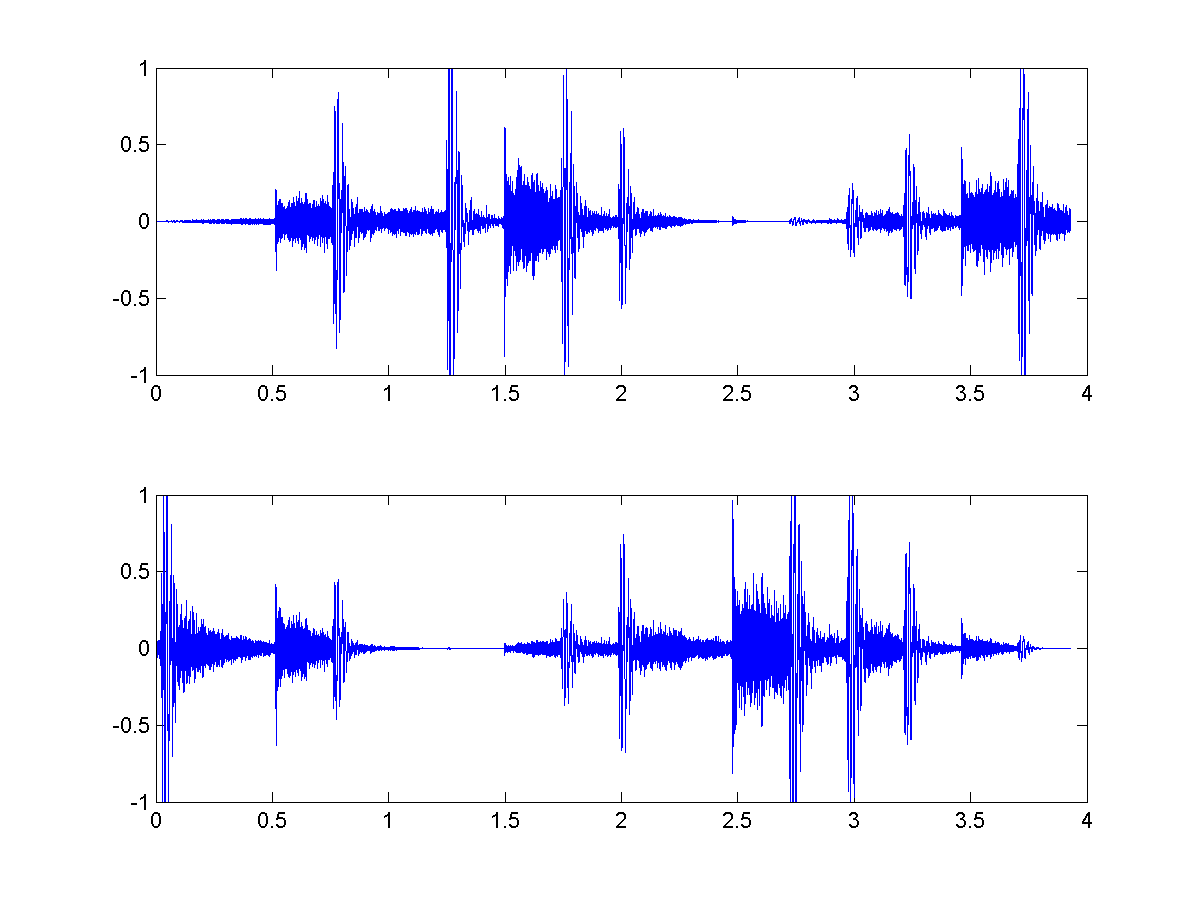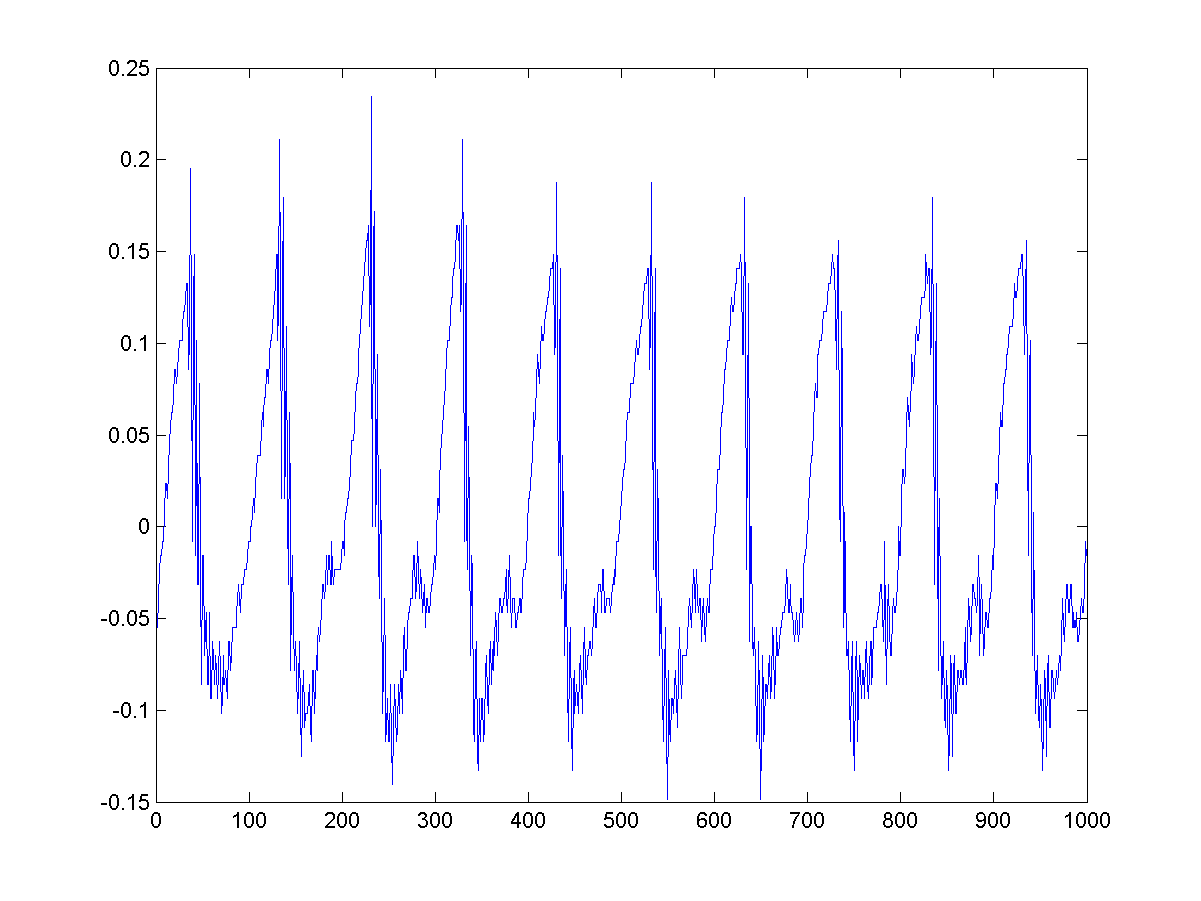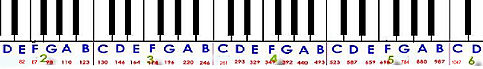300

Hint

Example 6: 20-音訊讀寫、錄製與播放/audioRead06.m[y, fs]=audioread('welcome.wav', [1, inf], 'native'); p=audioplayer(y, fs); play(p); % 播放此音訊 time=(1:length(y))/fs; % 時間軸的向量 plot(time, y); % 畫出時間軸上的波形 class(y) % 顯示 y 的資料型態 ans = uint8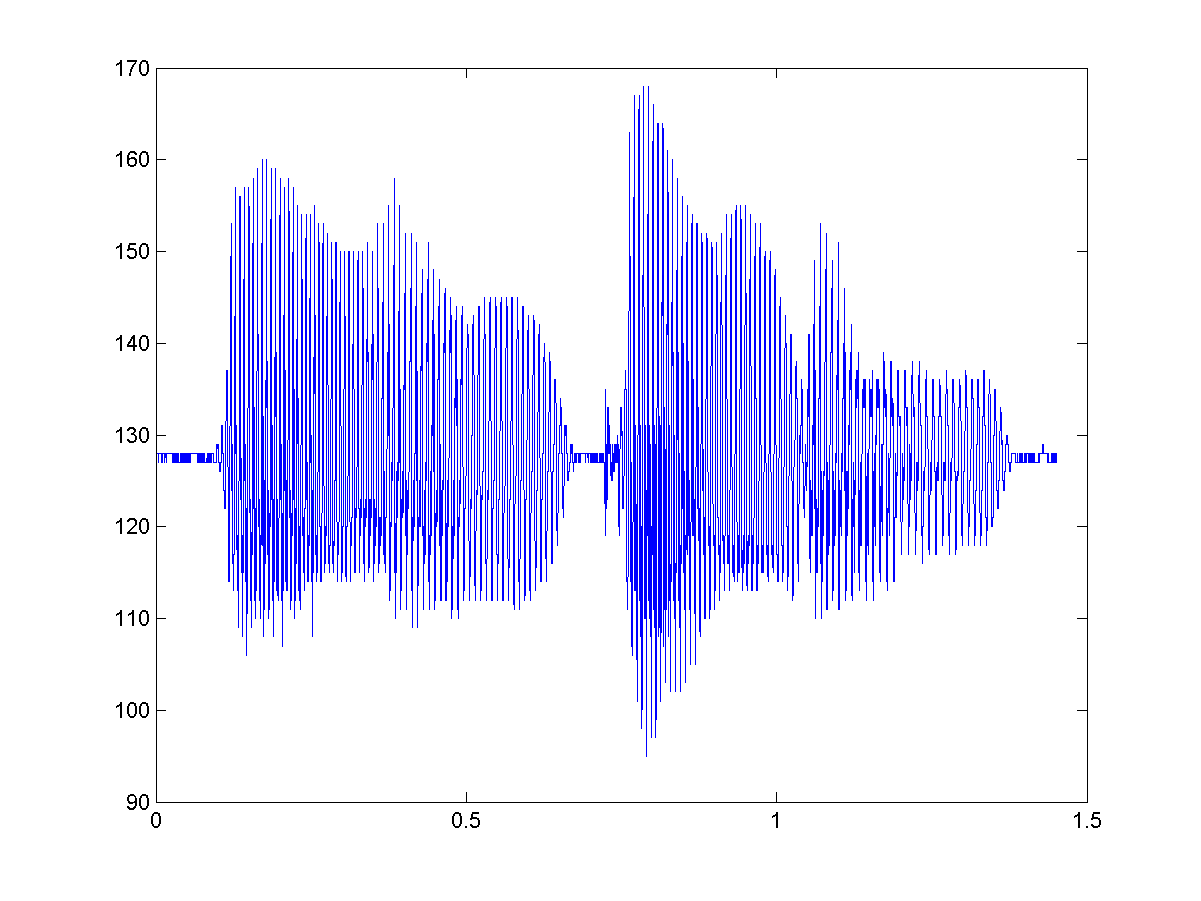MATLAB程式設計：入門篇